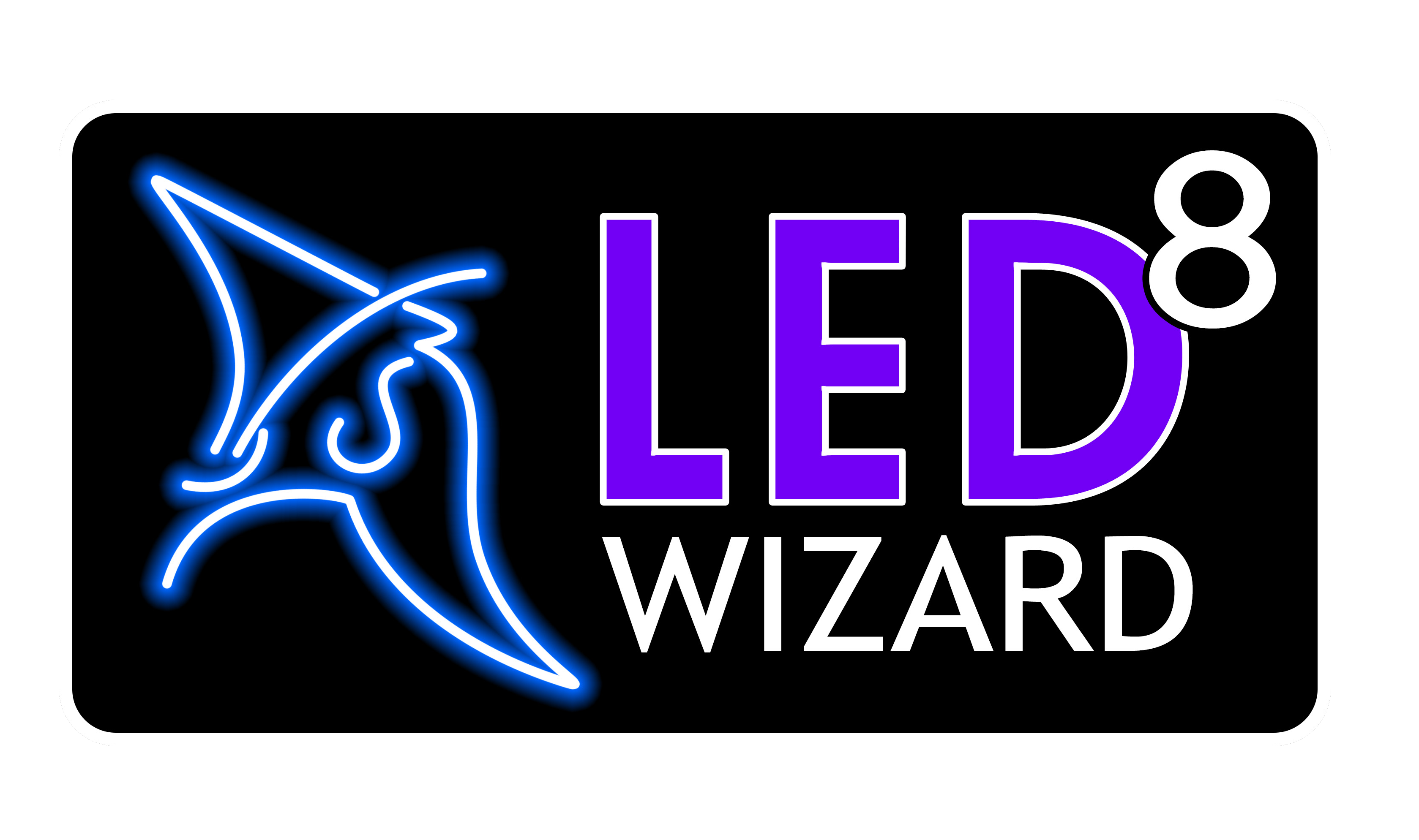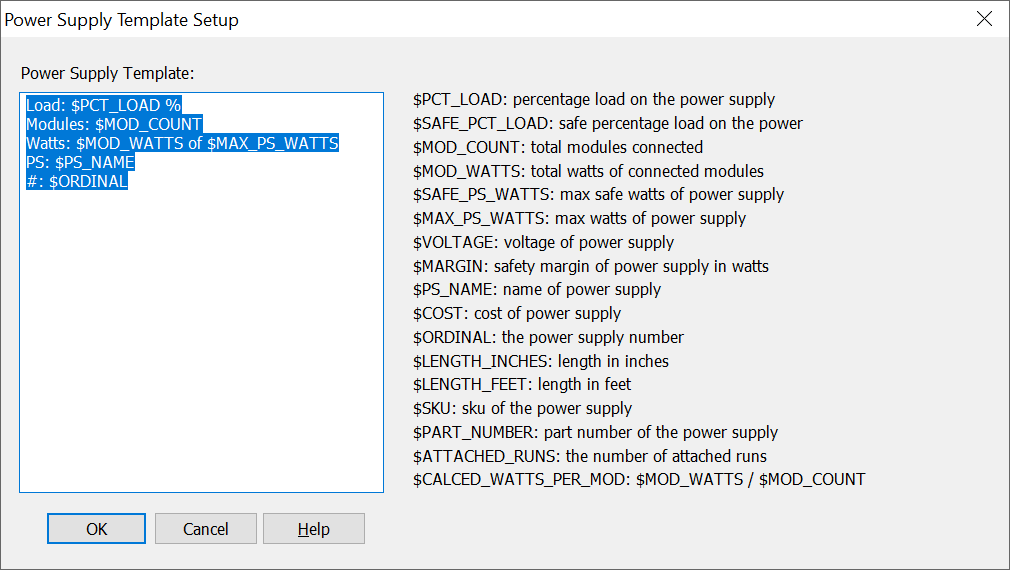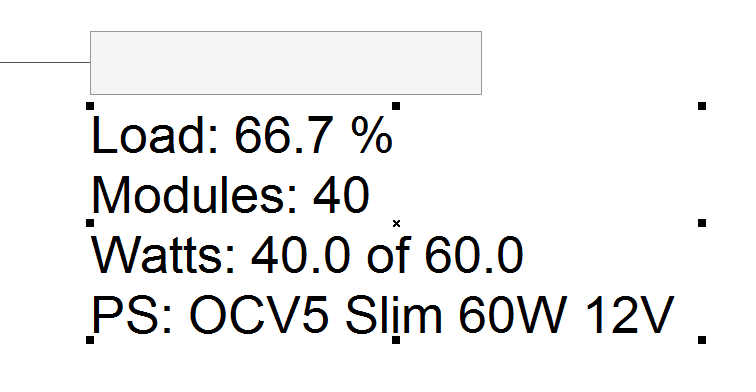LED Wizard 8 Documentation

## 8.10.23 Power Supply Template Setup

This dialog box lets you customize the power supply info text that appears beneath each power supply in your layouts.The large edit box on the left displays the template used when creating the power supply info text. You can rearrange the order and contents of each line as you wish.

The special variables that start with "\$" are replaced with the actual value. After the data variable name, you can add additional text labels, such as "%" in the first line for the load percentage.Please note that you can scale this power supply info by clicking on the text and then using the upper right control point. On larger layouts with many power supplies, sometimes this data can appear too small. The retangular box is the the actual size of the power supply and cannot be scaled.Below is a description of the variables:

This is the percentage load of the modules on the power supply, that is, the total watts divided by the total watts (not the safe watts) x 100.

This is the safe percentage load of the modules on the power supply, that is, the total watts divided by the total safe watts x 100.

##### \$MOD_COUNT

This is the total number of modules connected to the power supply.

##### \$MOD_WATTS

This is the total watts of all the modules connected to the power supply. It's simply the number of modules times the watts per module.

##### \$SAFE_PS_WATTS

This is the total safe watts of the power supply, which is the total watts minus the safety margin in watts.

##### \$MAX_PS_WATTS

This is the total watts of the power supply.

##### \$VOLTAGE

This is the voltage of this power supply, usually 12 or 24.

##### \$MARGIN

This is the safety margin of this power supply in watts.

##### \$LENGTH_INCHES

This is the total length of the attached modules in a Flex layout, in inches.

##### \$PS_NAME

This is the name of the power supply.

##### \$COST

This is the cost of this power supply.

##### \$ORDINAL

This is the sequential number of the power supply.

##### \$SKU

This is the SKU of the power supply.

##### \$PART_NUMBER

This is the part number of the power supply. A given power supply may or may not have a SKU and Part Number.

##### \$ATTACHED_RUNS

This will list the run numbers that are attached to that power supply, separated by commas. This should be used in conjunction with Show Run Numbers in the Options Menu.

##### \$CALCED_WATTS_PER_MOD: \$MOD_WATTS / \$MOD_COUNT

This is a calculation of the Watts per Module, based on the actual module watts divided by the total module count.